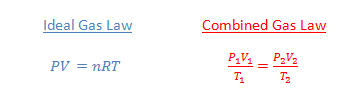## Balancing simple chemical reactions

When balancing equations, start with the atoms that show up in only one compound on each side. Figure out those coefficients first, and then work back and forth from the reactants side to the products side to find the coefficients of each of the other compounds.

Example 1: Balance the following equation:

… C5H12 + … O2 → … CO2 + … H2O

Solution: This reaction showing the combustion of pentane is not too complicated. Notice that carbon appears in only one compound on each side, so it’s a good place to start. The five carbon atoms on the left need to be balanced by five carbon atoms on the right:

1 C5H12 + … O2 → 5 CO2 + … H2O

There are 12 hydrogen atoms on the left, so balance these on the right:

1 C5H12 + … O2 5 CO2 + 6 H2O

Finally, count up all the oxygen atoms on the right (there are 16 of them!) and balance with the O2 on the left:

1 C5H128 O2 5 CO2 + 6 H2O

Example 2: Balance the following equation:

… C6H14 + … O2 → … CO2 + … H2O

Solution: Changing the pentane to hexane has made this equation more complicated. Let’s see why. First, let’s balance the carbon and hydrogen as before:

1 C6H14 + … O2 6 CO27 H2O

There are now 19 oxygen atoms on the right side. How do you balance this on the left side? Some teachers will let you use fractions for your coefficients, and 19/2 is the correct fraction in this case. But most teachers want you to use only whole numbers for the coefficients. If you encounter a fraction, you need to multiply the entire equation by the denominator to finish the problem. Here, we multiply the equation above by 2:

2 C6H14 + … O2 12 CO2 + 14 H2O

Notice there are now 38 oxygen atoms on the right side, and the last step is simple:

2 C6H14 + 19 O2 12 CO2 + 14 H2O

Here’s a trick you can apply when the equation is a double replacement reaction:

Example 3: Balance the following reaction:

… Na2SO4 + … Al(NO3)3 → … NaNO3 + … Al2(SO4)3

Solution: Let’s start by balancing the aluminum:

… Na2SO4 + 2 Al(NO3)3 → … NaNO3 + 1 Al2(SO4)3

Now instead of balancing the sulfur atoms, we can save time by balancing the sulfate ions instead. We treat them as a group rather than as separate atoms. There are three sulfates on the right side, so we need three on the left:

3 Na2SO4 + 2 Al(NO3)3 → … NaNO3 + 1 Al2(SO4)3

Note that you can only balance polyatomic ions like this when the ions do not change from reactant side to product side. Finally, you can see there are six sodium atoms on the left (or, six nitrate ions), so the final answer is:

3 Na2SO4 + 2 Al(NO3)3 6 NaNO3 + 1 Al2(SO4)3

## Figuring out conversion factors

A conversion factor expresses the relationship between the unit in the numerator and the unit in the denominator. The quantities are actually the same, but the numbers are different because the units are different. Any time you can express a fixed relationship between two quantities, you can make two conversion factors out of that relationship. (Flipping the original conversion factor upside down gives you the second one!)

Examples

•  2.54 cm = 1 in. so the conversion factor is:$\dfrac {2.54 \, cm}{1 \, in} \text{ or } \dfrac{1 \, in}{2.54 \, cm}$

•   1.00 cal = 4.184 J, so the conversion factor is:$\dfrac {1.00 \, cal}{4.184 \, J} \text{ or } \dfrac{4.184 \, J}{1.00 \, cal}$

•  1.00 mole O2 has a mass of 15.999 g, so the conversion factor is:$\dfrac {15.999 \, g \text{ O}_2}{1.00 \, mol \text{ O}_2} \text{ or } \dfrac{1.00 \, mol \text{ O}_2}{15.999 \, g \text{ O}_2}$

• In the production of ammonia, N2 + 3 H2 → 2 NH3, two moles of ammonia are produced for every 3 mol of H2 consumed, so the conversion factor is:$\dfrac {2 \, mol \text{ NH}_3}{3 \, mol \text{ H}_2} \text{ or } \dfrac{3 \, mol \text{ H}_2}{2 \, mol \text{ NH}_3}$

## Ideal Gas Law vs. Combined Gas Law

When you are doing gas law problems, your first decision should be whether to use the Ideal gas law or the combined gas law. How do you know which to use?The Ideal Gas Law is used when you have one set of conditions for your system. Then if you know any three of the values for pressure, volume, moles and temperature, you can solve for the unknown quantity. The Combined Gas Law is used when you have two sets of conditions. In other words, you know the initial pressure, volume and temperature, and two of those quantities change to a new set of conditions. Then you can calculate the new value of the third quantity.

Example 1: What is the volume of a container that holds 0.561 mol of a gas at 1.15 atm at 27°C?

Answer: This problem describes a single set of conditions, so use the Ideal Gas Law:$PV=nRT$$(1.15 \, atm)(V)=(0.561 \, mol) \! \left ( 0.0821 \, \frac{\ell \cdot atm}{mol \cdot K} \right ) \! (300 \, K)$$V=12.0 \, L$

Example 2: A 3.00 L container holds an ideal gas at STP. What will the new pressure be if the volume is increased to 3.75 L and the temperature is increased to 500 K?

Answer: This problem describes an initial condition and a new set of conditions, so use the combined gas law:$\dfrac{P_1V_1}{T_1}= \dfrac{P_2V_2}{T_2}$$\dfrac{(1.00 \, atm)(3.00 \, L)}{273 \, K}= \dfrac{(P)(3.75 \, L)}{500 \, K}$$P=1.47 \, atm$